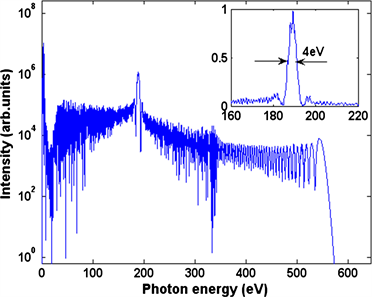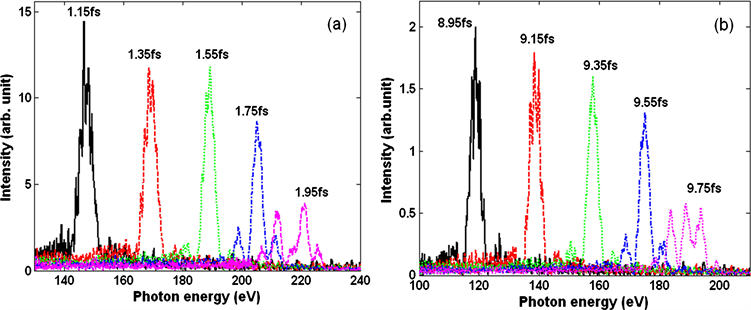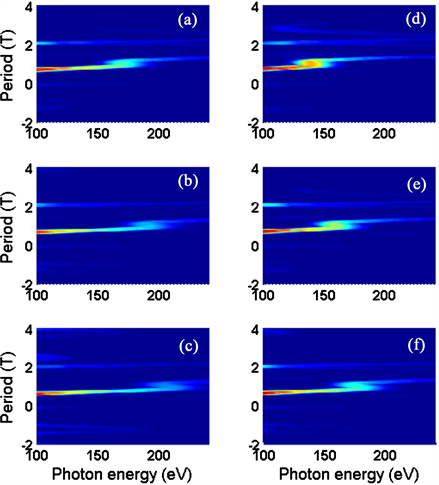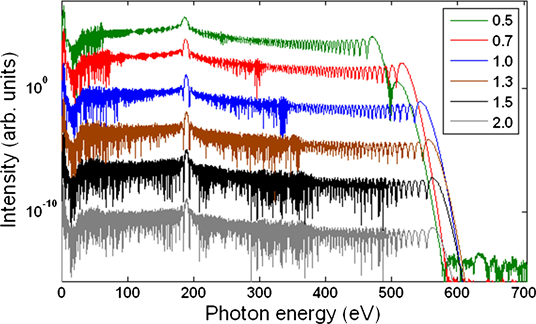﻿ 多周期强激光场中高次谐波选择性增强

# 多周期强激光场中高次谐波选择性增强Control of Enhanced High-Order Harmonic Generation in a Multi-Cycle Laser Field

Abstract: By using strong-field approximation, we theoretically investigate the selective enhancement of high-order harmonic generation in a small spectral range driven by a multi-cycle three-color laser field which is composed of an 800 nm laser pulse, a 1600 nm laser pulse and a 2400 nm laser pulse. The results show that a narrow-bandwidth spectrum can be selectively enhanced with the intensity increased by nearly one order of magnitude compared to the adjacent harmonics, which can be attributed to the modified electron trajectories in the three-color field. Furthermore, it is revealed that the central wavelength of the enhanced spectrum can be effectively tuned by changing the time delay between laser pulses of different colors.

1. 引言

2. 理论模型

$\begin{array}{l}x\left(t\right)=i{\int }_{0}^{\infty }\text{d}\tau \left(\frac{\pi }{\epsilon +i\tau /2}\right){d}_{x}^{*}\left({p}_{st}\left(t,\tau \right)-{A}_{x}\left(t\right)\right){d}_{x}\left({p}_{st}\left(t,\tau \right)-{A}_{x}\left(t-\tau \right)\right)\\ \text{}×E\left(t-\tau \right)\mathrm{exp}\left[-i{S}_{st}\left(t,\tau \right)\right]\mathrm{exp}\left(-{\int }_{-\infty }^{t}w\left({t}^{\prime }\right)\text{d}{t}^{\prime }\right)+c.c\end{array}$ (1)

${p}_{st}\left(t,\tau \right)={\int }_{t-\tau }^{t}\text{d}{t}^{\prime }A\left({t}^{\prime }\right)/\tau$ (2)

${S}_{st}\left(t,\tau \right)={I}_{p}-\frac{1}{2}{p}_{st}^{2}\left(t,\tau \right)+\frac{1}{2}{\int }_{t-\tau }^{t}\text{d}{t}^{\prime }{A}_{x}^{2}\left({t}^{\prime }\right)$ (3)

d为跃迁矩阵元，本文中采用类氢原子的跃迁矩阵元，表达式为

$d\left(p\right)=i\frac{{2}^{7/2}{\left(2{I}_{p}\right)}^{5/4}}{\pi }\frac{p}{{\left({p}^{2}+2{I}_{p}\right)}^{3}}$ (4)

${I}_{p}$ 为电离势。

3. 窄带高次谐波增强及分析

$\begin{array}{l}{E}_{s}={E}_{1}\mathrm{exp}\left[-2\mathrm{ln}\left(2\right)\cdot {t}^{2}/{\tau }_{1}^{2}\right]\cdot \mathrm{cos}\left({\omega }_{1}t\right)+{E}_{2}\mathrm{exp}\left[-2\mathrm{ln}\left(2\right)\cdot {t}^{2}/{\tau }_{2}^{2}\right]\cdot \mathrm{cos}\left({\omega }_{2}t\right)\\ \text{}+{E}_{3}\mathrm{exp}\left[-2\mathrm{ln}\left(2\right)\cdot {\left(t+{T}_{d}\right)}^{2}/{\tau }_{3}^{2}\right]\mathrm{cos}\left({\omega }_{3}\left(t+{T}_{d}\right)\right)\end{array}$ (5)

3.1. 延迟时间对增强谱段的影响Figure 1. High-order harmonic spectrum driven by the laser field synthesized by a 16 fs, 800 nm pulse, a 32 fs, 1600 nm pulse and a 48 fs, 2400 nm laser pulse. Inset: The enhanced harmonic spectrum in a linear scaleFigure 2. The enhanced high-order harmonic spectra at different time delays: (a) 1.15 fs~1.95 fs and (b) 8.95 fs~9.75 fsFigure 3. Time-frequency analyses corresponding to high-order harmonic spectrum in Figure 2 at the time delays of (a) 1.35 fs, (b) 1.55 fs, (c) 1.75 fs, (d) 9.15 fs, (e) 9.35 fs, (f) 9.55 fs.

3.2. 脉冲宽度对增强谱段的影响Figure 4. High-order harmonic spectrum driven by three-color field of the different durations (R = 0.5~2.0)

4. 结论

NOTES

*通讯作者。

 Krausz, F. and Ivanov, M. (2009) Attosecond Physics. Reviews of Modern Physics, 81, 163.
https://doi.org/10.1103/RevModPhys.81.163

 Jin, C., Wang, G., Wei, H., et al. (2014) Waveforms for Optimal Sub-KeV High-Order Harmonics with Synthesized Two- or Three-Colour Laser Fields. Nature Communications, 5, 4003.
https://doi.org/10.1038/ncomms5003

 Chini, M., Zhao, K. and Chang, Z. (2014) The Generation, Characterization and Applications of Broadband Isolated Attosecond Pulses. Nature Photonics, 8, 178-186.
https://doi.org/10.1038/nphoton.2013.362

 Corkum, P.B. (1993) Plasma Perspective on Strong Field Multiphoton Ionization. Physical Review Letters, 71, 1994-1997.
https://doi.org/10.1103/PhysRevLett.71.1994

 Schafer, K.J., Yang, B., DiMauro, L.F. and Kulander, K.C. (1993) Above Threshold Ionization beyond the High Harmonic Cutoff. Physical Review Letters, 70, 1599-1602.
https://doi.org/10.1103/PhysRevLett.70.1599

 Yu, Y., Song, X., Fu, Y., et al. (2008) Theoretical Investigation of Single Attosecond Pulse Generation in an Orthogonally Polarized Two-Color Laser Field. Optics express, 16, 686-694.
https://doi.org/10.1364/OE.16.000686

 Yuan, H., He, L., Wang, F., et al. (2017) Generation of Isolated Attosecond Pulses in a Multi-Cycle Inhomogeneous Two-Color Field without CEP Stabilization. Optical and Quantum Electronics, 49, 214.
https://doi.org/10.1007/s11082-017-1048-x

 葛鑫磊. 少周期激光脉冲附加太赫兹场对高次谐波发射的影响[J]. 原子与分子物理学报, 2018(35): 993-998.

 Kim, I.J., Kim, C.M., Kim, H.T., et al. (2005) Highly Efficient High-Harmonic Generation in an Orthogonally Polarized Two-Color Laser Field. Physical Review Letters, 94, Article ID: 243901.
https://doi.org/10.1103/PhysRevLett.94.243901

 Goulienlmakis, E., Schultze, M., Hofstetter, M., et al. (2008) Single-Cycle Nonlinear Optics. Science, 320, 1614-1617.
https://doi.org/10.1126/science.1157846

 Bartels, R., Backus, S., Zeek, E., et al. (2000) Shaped-Pulse Optimization of Coherent Emission of High-Harmonic Soft X-Rays. Nature, 406, 164-166.
https://doi.org/10.1038/35018029

 Wei, P., Miao, J., Zeng, Z., et al. (2013) Selective Enhancement of a Single Harmonic Emission in a Driving Laser Field with Subcycle Waveform Control. Physical review letters, 110, Article ID: 233903.
https://doi.org/10.1103/PhysRevLett.110.233903

 Zeng, Z., Cheng, Y., Fu, Y., et al. (2008) Tunable High-Order Harmonic Generation and the Role of the Folded Quantum Path. Physical Review A, 77, Article ID: 023416.
https://doi.org/10.1103/PhysRevA.77.023416

 Yao, J., Cheng, Y., Chen, J., et al. (2011) Generation of Narrow-Bandwidth, Tunable, Coherent XUV Radiation Using High-Order Harmonic Generation. Physical Review A, 83, Article ID: 033835.
https://doi.org/10.1103/PhysRevA.83.033835

 Peng, D., Pi, L.W., Frolov, M.V., et al. (2017) Enhancing High-Order-Harmonic Generation by Time Delays between Two-Color, Few-Cycle Pulses. Physical Review A, 95, Article ID: 033413.
https://doi.org/10.1103/PhysRevA.95.033413

 Brabec, T. and Krausz, F. (2000) Intense Few-Cycle Laser Fields: Frontiers of Nonlinear Optics. Reviews of Modern Physics, 72, 545-591.
https://doi.org/10.1103/RevModPhys.72.545

Top